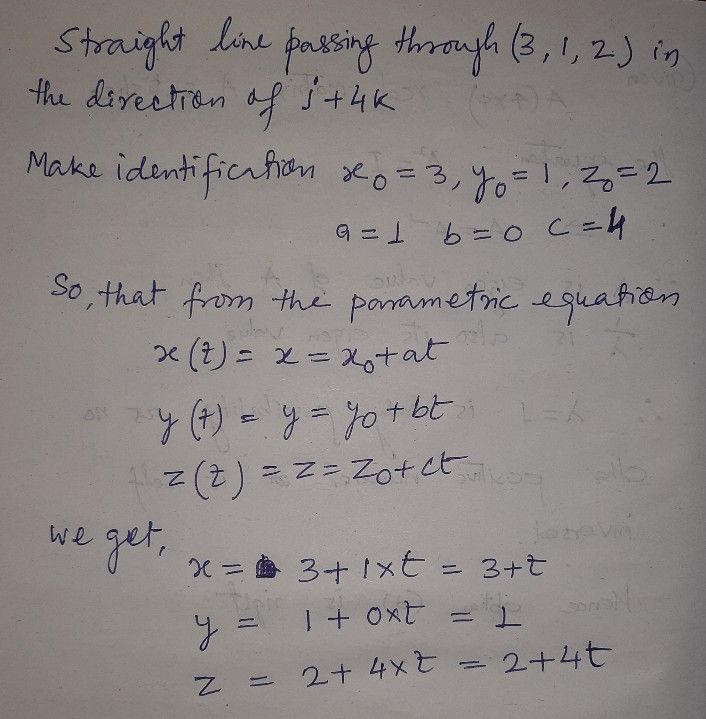Symbol
Problem.Find the parametric representation of the straight line passing through $\left(3$ $1,2\right)$ in the direction of $144k$ $44$ $a$
Calculus
Search count: 112
SolutionQanda teacher - Anisha